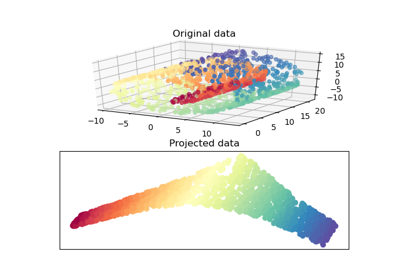# sklearn.manifold.locally_linear_embedding¶

sklearn.manifold.locally_linear_embedding(X, *, n_neighbors, n_components, reg=0.001, eigen_solver='auto', tol=1e-06, max_iter=100, method='standard', hessian_tol=0.0001, modified_tol=1e-12, random_state=None, n_jobs=None)[source]

Perform a Locally Linear Embedding analysis on the data.

Read more in the User Guide.

Parameters
X{array-like, NearestNeighbors}

Sample data, shape = (n_samples, n_features), in the form of a numpy array or a NearestNeighbors object.

n_neighborsinteger

number of neighbors to consider for each point.

n_componentsinteger

number of coordinates for the manifold.

regfloat

regularization constant, multiplies the trace of the local covariance matrix of the distances.

eigen_solverstring, {‘auto’, ‘arpack’, ‘dense’}

auto : algorithm will attempt to choose the best method for input data

arpackuse arnoldi iteration in shift-invert mode.

For this method, M may be a dense matrix, sparse matrix, or general linear operator. Warning: ARPACK can be unstable for some problems. It is best to try several random seeds in order to check results.

denseuse standard dense matrix operations for the eigenvalue

decomposition. For this method, M must be an array or matrix type. This method should be avoided for large problems.

tolfloat, optional

Tolerance for ‘arpack’ method Not used if eigen_solver==’dense’.

max_iterinteger

maximum number of iterations for the arpack solver.

method{‘standard’, ‘hessian’, ‘modified’, ‘ltsa’}
standarduse the standard locally linear embedding algorithm.

see reference 

hessianuse the Hessian eigenmap method. This method requires

n_neighbors > n_components * (1 + (n_components + 1) / 2. see reference 

modifieduse the modified locally linear embedding algorithm.

see reference 

ltsause local tangent space alignment algorithm

see reference 

hessian_tolfloat, optional

Tolerance for Hessian eigenmapping method. Only used if method == ‘hessian’

modified_tolfloat, optional

Tolerance for modified LLE method. Only used if method == ‘modified’

random_stateint, RandomState instance, default=None

Determines the random number generator when solver == ‘arpack’. Pass an int for reproducible results across multiple function calls. See :term: Glossary <random_state>.

n_jobsint or None, optional (default=None)

The number of parallel jobs to run for neighbors search. None means 1 unless in a joblib.parallel_backend context. -1 means using all processors. See Glossary for more details.

Returns
Yarray-like, shape [n_samples, n_components]

Embedding vectors.

squared_errorfloat

Reconstruction error for the embedding vectors. Equivalent to norm(Y - W Y, 'fro')**2, where W are the reconstruction weights.

References

1

Roweis, S. & Saul, L. Nonlinear dimensionality reduction by locally linear embedding. Science 290:2323 (2000).

2

Donoho, D. & Grimes, C. Hessian eigenmaps: Locally linear embedding techniques for high-dimensional data. Proc Natl Acad Sci U S A. 100:5591 (2003).

3

Zhang, Z. & Wang, J. MLLE: Modified Locally Linear Embedding Using Multiple Weights. http://citeseerx.ist.psu.edu/viewdoc/summary?doi=10.1.1.70.382

4

Zhang, Z. & Zha, H. Principal manifolds and nonlinear dimensionality reduction via tangent space alignment. Journal of Shanghai Univ. 8:406 (2004)

## Examples using sklearn.manifold.locally_linear_embedding¶